### 6. Arrays

#### By Joker. February 18th, 2020. 2:20 PM

##### Check the guide's index here.
Objectives
• What is an Array
• Array declaration
• Initializing arrays
• Indexes and element positions in an array
• Matrixes and multidimensional arrays
• Defining constants in C
• Differences between const and #define
• Generating random numbers

### Intro

After learning about C's basic data types (char, int, float and double) and its control structures (if, switch, while, for and do ... while), we're now going to study the way how we can process sets of data/values of the same type.

An array is nothing more than a set of consecutive elements, all of the same data type, which can be accessed individually from a single name.

As examples of using arrays, we can present:

1. Set of monthly comissions associated to a certain employee throughout the span of a year.
 12 000 5 000 2 300 1 230 7 400 ...
• Array of Mr. Justin's Comissions (Simple Integer Array).
• Each position corresponds to the value received in that month (Ex: 12 000 - january, 2 300 - march, etc.).

This way, you avoid declaring a variable for each month (12 variables on total), placing all information in one single variable.

2. Tic Tac Toe (Two Dimensional Character Array)
 X O X O

### Declaring Arrays

An array in C is declared the same way as a simple variable.

`int n;     /* Declaration of the variable n */`

Single dimension array declarations obey the following syntax:

type variable_name[elem_num]
• type - corresponds to the data type of each of the array's elements.
• variable_name - indicates the name by which that array will be recognized as.
• elem_num - constant value which indicates how many elements that array has.
Note: An array can contain elements of any data type. However, the array's elements are all of the same type, which is defined in the array's own declaration.

To declare an array with 20 integers:

`int g;       /* g is an array with 20 integers */`

To declare an array with 100 floating point numbers:

`float rent; /* rent is an array with 100 integers */`

Every element of an array can be identified by the same name (g or rent, in the previous examples), however, in order to identify each of them individually, you need a number (index) to indicate what is its position in the array.

In a declaration like

`float rent; /* rent is an array with 100 integers */`
• float - Type of each of the array's elements.
• 100 - Number of elements in the array.
• rent - The array's name.
• rent[i] - Value stored in the rent array's i position.

Let us suppose, then, that we pretended to declare an array with six integers, named array.

`int array;`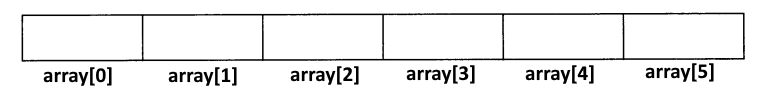Note: In C, the indexes of a n sized array always vary from 0 and n-1.

This way, each of the our array's six positions can be accessed by placing the respective index inbetween square brackets [].

Note: The first element's index, in any C array, is always 0 (ZERO).

Example: Place the value 123 in the array's first position.

`array = 123;`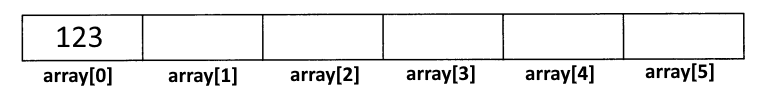Place the double of the array's first element's value on the last position.

`array = array*2;`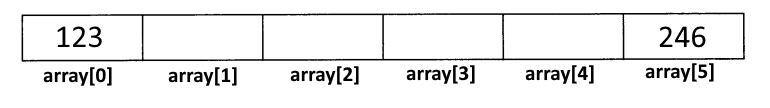Place the sum of the first and last elements on the array's third element.

```array = array + array;

/* Notice that the third element is contained on the index 2 position,
* because the array's elements start on the index 0
*/```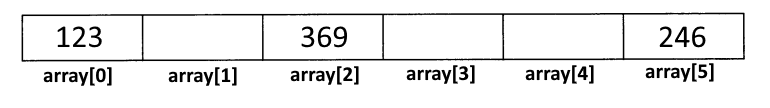Note: In an array, the n-th element is always on the position n - 1.

The position occupied by an array's element is also called that array element's index.

Initiate the array again, placing, in each position, that position's corresponding index.

```for(i = 0; i < 6; i++)
array[i] = i;```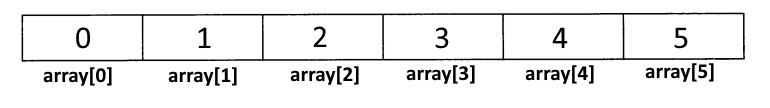An element's index can be represented by any expression that returns an integer value.

```array = 2;                /* 1 is an integer */
array[3 - 1] = 23;           /* equivalent to array = 23 */
array[1 + array] = 513;   /* equivalent to array[1 + 2] = 513 */
/* because array is an integer */```

### Automatically Initializing Arrays

Note: Just like variables, when arrays are created, they contain random values (GARBAGE) in each of its positions.

It's possible to automatically initialize every element in an array through following syntax:

type var[n] = {value_1, value_2, ..., value_n};

Example: Declare and initiate an array with all vowels in the alphabet.

`char vowel = {'a', 'e', 'i', 'o', 'u'};`

This way, we avoid having to write more code than needed:

```char vowel;
vowel = 'a';
vowel = 'e';
vowel = 'i';
vowel = 'o';
vowel = 'u';```
Note: If an array is declared with n elements and are placed only k values (k < n) in the array's initialization, then the array's k first elements will be initiated with the respected values, and the remaining ones will be initiated with the value ZERO.

Let's suppose the following declaration:

`int v = {10,20,30};`

In the previous example, the array's three first elements (indexes 0, 1 and 2) are initiated with the values 10, 20 and 30, respectively, and all other elements are initiated with the value 0.

Thus, the following instructions are equivalent

```int v = {10, 20, 30};
int v = {10, 20, 30, 0, 0, 0, 0, 0, 0, 0};```
Attention: An array's positions are only initiated if its declaration is followed by = { value_1, ...., value_n };

Example: Write a program that reads the wages paid to an individual during a whole year. Next, the program should show the monthly values and the annual total.

prog0601.c

``` 1: #include <stdio.h>
2:
3: int main(){
4:   float wag; /* 12 months */
5:   float total;
6:   int i;
7:
8:   for(i = 0; i < 12; i++){
9:     printf("Input the %dth month's wage: ", i+1);
10:     scanf("%f", &sal[i]);
11:   }
12:
13: /* Show the monthly values and calculate the total */
14:   puts(" Month       Value ");
15:   for(i = 0, total = 0.0; i < 12; i++){
16:     printf(" %5d       %9.2f\n", i+1, sal[i]);
17:     total += sal[i];
18:   }
19:
20:   printf("Annual Total: %9.2f\n", total);
21:   return 0;
22: }```

```\$ ./prog0601
Input the 1th month's wage:1000
Input the 2th month's wage:2000
Input the 3th month's wage:3500
Input the 4th month's wage:6480.5
Input the 5th month's wage:32000
Input the 6th month's wage:4560.5
Input the 7th month's wage:10000
Input the 8th month's wage:7450
Input the 9th month's wage:6471
Input the 10th month's wage:1240
Input the 11th month's wage:1240
Input the 12th month's wage:1240
Month       Value
1       1000.00
2       2000.00
3       3500.00
4       6480.50
5      32000.00
6       4560.50
7      10000.00
8       7450.00
9       6471.00
10       1240.00
11       1240.00
12       1240.00
Annual Total:  77182.00```

Notice that the user doesn't need to know how the data is stored in our program. Therefore, when we ask the first month's wage (month 1), we'll have to store it in our array's 0 position. It doesn't make sense to solicit the user for the 0 month's wage. Because of that, we write the value i + 1 on screen and storing the value on the i index of the array.

``` 8:   for(i = 0; i < 12; i++){
9:     printf("Input the %dth month's wage: ", i+1);
10:     scanf("%f", &sal[i]);
11:   }```

The previous example can cause some confusion, because we're used to thinking of months as values between 1 and 12, and not between 0 and 11.

Because of that, some programmers solve the problem in another way.

They declare an array with 13 elements (indexes 0..12), in order to use the indexes relative to the pretended month (1...12). The 0 index is simply ignored.

prog0602.c

``` 1: #include <stdio.h>
2:
3: int main(){
4:   float wag; /* 12 months */
5:   float total;
6:   int i;
7:
8:   for(i = 1; i <= 12; i++){
9:     printf("Input the %dth month's wage: ", i+1);
10:     scanf("%f", &sal[i]);
11:   }
12:
13: /* Show the monthly values and calculate the total */
14:   puts(" Month       Value ");
15:   for(i = 1, total = 0.0; i <= 12; i++){
16:     printf(" %5d       %9.2f\n", i+1, sal[i]);
17:     total += sal[i];
18:   }
19:
20:   printf("Annual Total: %9.2f\n", total);
21:   return 0;
22: }```

An array's declaration and initialization can be done without indicating the array's number of elements.

type var[ ] = { value_1, value_2, ..., value_n };

In this case, the compiler will create an array with as many elements as there are initial values.

### Arrays - Declaration Examples

```int v;               /* Array with 10 non initiated elements    */
int v = {5, 10, 15};  /* Array with 3 automatically initiated
elements with the values 5, 10 and 15   */
int v = {5, 10, 15}; /* Array with 10 elements. The first three
are initiated with the values 5, 10 and
15. The rest are initiated with 0.      */
int v[] = {5, 10, 15};   /* Array with 3 elements initiated with the
values 5, 10 and 15.
Equivalent to int v = {5, 10, 15};   */
int v[];                 /* Incorrect declaration - causes a
Compiling Error                         */```

### Some Notes About Arrays

• An array's elements are always stored in contiguous memory positions.
• The elements of an array declared with no initialization contain random values.
• An array's first element index is always 0 (ZERO).
• An array's indexes with n elements always vary between 0 and n-1.
• The existent value in a position of the array a can be obtained through the index in which that position is stored a[index].
• The compiler does not verify if the indexes used in an array are correct or not. A common example of an error in manipulating an array with n elements is using the index n (v[n]), which does not belong to the array and can cause severe problems, because we're altering memory that does not belong to us.
• The compiler doesn not verify if the indexes used in an array are correct or not with the array's declaration. I.E., we can declare an array with a deimension of 3 and reference (wrongly) superior or inferior positions, and the compiler won't indicates any errors.
• An array can be automatically initiated with the value set existent between curly brackets, following the = symbol. This can only be done when you're declaring the array.
• If the number of initial values is lower than the number of elements in the array, the remaining elements will be initiated with the value ZERO.
• You can declare an array without indicating the number of elements it will contain, as long as they're placed in its initial charge. In this case, the compiler automatically calculates the number of elements the array will contain.
• You can't declare arrays with no dimensions. If we don't know the pretended dimension, how will the compiler know the necessary space to hold it in?

### Passing Arrays to Functions

Let's make a supposition out of the following declarations:

`int v;int x;`

Let's suppose, then, that our intention was to initiate the arrays v and x with the value ZERO in all positions and that we pretended to utilize a function to execute such an initialization.

Since the arrays have different dimensions, we'll have to eventually define two distinct functions (one for 10 integer arrays, another for 20 integer arrays).

Let's, then, write the code for each of them:

```void inic1(int s){
int i;
for(i = 0; i < 10; i++)
s[i] = 0;
}

void inic2(int s){
int i;
for(i = 0; i < 20; i++)
s[i] = 0;
}```

Now we have the code for each function.

Invoking these functions on main should be done like this:

```int main(){
int v;

inic1(v);   /* Initiate the array v using the function inic1 */
inic2(x);   /* Initiate the array x using the function inic2 */
return 0;
}```

Notice that if we send a 10 integer array to the function inic1, it should have a parameter of the same type as the variable.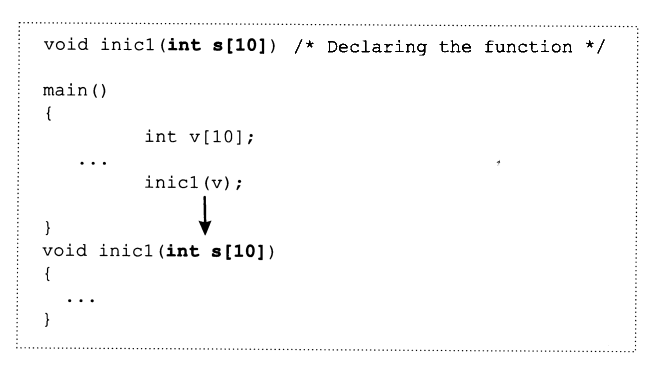However, the two initialization functions can be substituted by a single one that initiates any integer array with the value 0. This is possible because, in C, what matters is not the size of the array that is passed to a function, but yes its element type, and, in that case, both arrays are composed of integers.

Note: In C, you can't know how many elements were declared in a vector that was passed as an argument over to a function.

It is possible to write a function that initiates any integer array. However (because of the previous note), we have to indicate the number of elements the array contains.

prog0603.c

``` 1: #include <stdio.h>
2:
3: int void inic(int s[], int n){
4:   int i;
5:   for(i = 0; i < n; i++)
6:     s[i] = 0;
7: }
8:
9: int main(){
10:   int v;
11:   int x;
12:
13:   inic(v, 10);
14:   inic(x, 20);
15:   return 0;
16: }```

Notice, now, that the function void inic(int s[], int n) receives an integer array (without indicating its dimension) and an integer that indicates what's the number of elements to initiate.

This way, its possible to indicate an array of integers (whatever is the dimension with which it was declared), because we do not indicate in the function parameter how many elements the array contains.

Note: If we indicate the array size in the function parameter, the array's number of elements is simply ignored by the compiler. The function only cares about the array's element type. The size to consider is of the programmer's exclusive responsibility.

### Constants

Writing programs should be done in a way that a small change won't cause big transformations in its code.

Let's, then, suppose the following program:

prog0604.c

``` 1: #include <stdio.h>
2:
3: void inic(int s[]){
4:   int i;
5:   for(i = 0; i < 10; i++)
6:     s[i] = 0;
7: }
8:
9: int main(){
10:   int v, i;
11:
12:   inic(v);
13:
14:   for(i = 0; i < 10; i++)
15:     v[i] = i;
16:
17:   for(i = 10 - 1; i >= 0; i--)
18:     printf("%d\n", v[i]);
19:   return 0;
20: }```

This is a program that works for an array with 10 elements. However, if its alteration is extremely urgent, in order for it to be used not with 10, but with 25 integers, the solution is to alter all occurrences of the number 10 for number 25.

``` 5:   for(i = 0; i < 10; i++)
10:   int v, i;
14:   for(i = 0; i < 10; i++)
17:   for(i = 10 - 1; i >= 0; i--)```

Since it's a small program, the effort won't be much, but imagine if it was a program with thousands of lines of code.

In this case, we could use a good editor and automaticly alter all occurrences of 10 for 25 not much effort.

However, some unwanted effects could occur. What would happen to the following strip of code:

```if(grade >= 10)
printf("Approved");
else
printf("Disapproved");```

Most properly would turn into:

```if(grade >= 25)
printf("Approved");
else
printf("Disapproved");```

This would increase, in a significant way, the number of disapproved students in that subject.

The solution is in using constants that, once altered, propague their new value through all occurrences.

### Defining Constants

A constant is nothing more than a name correspondent to a fixed value (cannot be altered throughout the execution of a program).

These should be defined, normally, outside of any function, in order to make them visible to the entire program.

Note: Constants should be declared outside of functions, in order for them to be "visible" to all the code of the program. Normally, its definition is done immediately after the #includes lines.

Defining constants can be done in two distinct ways:

• Through the reserved keyword const;
const type symbol = value;
• Through the pre-processing directive #define
#define symbol value

Examples:

prog0605.c

``` 1: #include <stdio.h>
2: const int num = 10;
3:
4: void inic(int s[]){
5:   int i;
6:   for(i = 0; i < num; i++)
7:     s[i] = 0;
8: }
9:
10: int main(){
11:   int v[num], i;
12:
13:   inic(v);
14:   for(i = 0; i < num; i++)
15:     v[i] = i;
16:   for(i = num - 1; i >= 0; i--)
17:     printf("%d\n", v[i]);
18:   return 0;
19: }```

prog0606.c

``` 1: #include <stdio.h>
2: #define NUM 10          /* No Semi-Colon */
3:
4: void inic(int s[]){
5:   int i;
6:   for(i = 0; i < NUM; i++)
7:     s[i] = 0;
8: }
9:
10: int main(){
11:   int v[NUM], i;
12:
13:   inic(v);
14:   for(i = 0; i < NUM; i++)
15:     v[i] = i;
16:   for(i = NUM - 1; i >= 0; i--)
17:     printf("%d\n", v[i]);
18:   return 0;
19: }```

If we want to alter our array size from 10 to 25, we'll only need to alter the line where the constant is defined, independently of its number of occurrences inside of the program.

### Differences between const and #define

• A constant defined with const exists physically in a determined position of memory.
• A constant defined with #define does not exist physically in memory, and its value is substituted throughout the program in the pre-processing phase (even before compiling).
• The keyword const makes part of the reserved words in C.
• The keyword #define is a directive that indicates the pre-processor that the following symbol will keep the value following said symbol. The pre-processor, before compiling the program, substitutes all occurrences of the symbol by the value with which it was defined. (In the previous example, it substitutes all occurrences of NUM with 10). It's like there's a little goblin substituting all occurrences of NUM with 10, immediately before the program's compilation.
• A constant defined with the word const stays with the type that was placed on its definition.
• The type associated to a constant defined with #define is the type that results from the expression that appears in the value component.
• Since the #define keyword does not make part of the C language, this line is not followed by a semi-colon.
Note: Constants defined with the symbol #define are called Symbolic Constants. Although it's not mandatory, C programmers usually declare symbolic constants in uppercase.

### Matrixes and Multidimensional Arrays

Up until this moment, we could only work with unidimensional arrays. Let's, now, learn how to use arrays with more than one dimension.

Note: There are no limits to the number of dimensions that an array can have.

Declaring unidimensional arrays is nothing more than a particular case in declaring arrays with any number of dimensions.

Declaring an array with n dimensions is done through the following syntax:

type array[dim_1] [dim_2] [...] [dim_n]

As an example, let's observe how we could implement the famous game Tic Tac Toe, through an array as a game board.

 X O X O

As you can see, we need a two dimensional array (vulgarly called a Matrix).

Each square will have an X, an O or a blank space. Thus, it's a character array.

The declaration should be done like this:

```#define DIM 3
........
char tic[DIM][DIM]; /* 3 Rows, each with 3 Positions```
Note: In C, an array declared with two dimensions is not really a matrix, it's actually an array of arrays. The same applies to arrays with a dimension higher than 2.

In the previous case, what we've declared is not a matrix, but yes an array with three positions tic where, in each of those positions, there's a three character array.

 tic ==> X O tic ==> X tic ==> O

Thus:

• tic - it's a character array with two dimensions 3 x 3.
• tic[i] - it's a three character array.
• tic[i][j] - character stored in the i row and j column of the tic array.

If we declare an array

`int v;`
• v - it's a three element array (each element is a 4 integer array),
• v[i] - it's a four integer array.
• v[i][j] - integer stored in the i,j position of the v array.

### Multidimensional Arrays: Automatic Initialization

The initialization must be done in the same way as with unidimensional arrays.

Let's suppose, then, that we wanted to start off our game of tic tac toe with blank spaces on all positions.

`char tic[DIM][DIM] = {' ',' ',' ',' ',' ',' ',' ',' ',' '};`

Although this is the traditional way to initialize the array, it is not correct, because tic is not a 12 element array - it's an array made out of three arrays.

The initializing of a one dimensional array with three spaces is done like this:

`char v = {' ',' ',' '};`

Now, since the initialization of an array is done through curly brackets, the initialization of the tic array should be done like this:

`char tic[DIM][DIM] = {.......};`

Since the tic array is a three element array (each one is also an array), then we'll have to place only three elements between curly brackets:

`char tic[DIM][DIM] = {  ,  ,  };`

Our current problem is knowing what elements to place.

Question: What corresponds to tic?

Answer: tic is a 3 character array?

Question: How do you initiate a 3 character array with blank spaces?

Answer: By placing the respecive characters between curly brackets {' ',' ',' '}?

Now we know how to initiate the first element of the array.

`char tic[DIM][DIM] = {{' ',' ',' '},  ,  };`

The others are initiated in the same way.

Thus, the initialization of the tic array can be done in two distinct ways:

`char tic[DIM][DIM] = {' ',' ',' ',' ',' ',' ',' ',' ',' '};`

or

`char tic[DIM][DIM] = {{' ',' ',' '},{' ',' ',' '},{' ',' ',' '}};`

Example: Declare and initiate the integer array v with any integer values you want.

`int v = {{1,2,3,4},{5,6,7,8}};`
Note: The indexes of an array's every dimension always start at 0 (ZERO).

Exercise:

Write a program that sets the game board for Tic-Tac-Toe as follows, after being initiated with blank spaces during its declaration.

 X O X O

Next, print it out on-screen.

test.c

``` 1: #include <stdio.h>
2: #define DIM 3
3:
4: int main(){
5:   char tic[DIM][DIM] = {{' ',' ',' '},{' ',' ',' '},{' ',' ',' '}};
6:   int i, j;
7:
8:   tic = 'X';
9:   tic = 'X';
10:   tic = 'O';
11:   tic = 'O';
12:
13:   for(i = 0; i < DIM; i++){
14:     for(j = 0; j < DIM; j++)
15:       printf("%c %c", tic[i][j], j == DIM - 1 ? ' ' : '|');
16:     if(i != DIM - 1) printf("\n--------\n");
17:   }
18:   return 0;
19: }```

```\$ ./test
X |  |O
--------
|X |
--------
|  |O ```

In the automatic initialization of an array, you can only omit a numeric value for the dimension furthest on the left, the respective value being calculated by the compiler.

```chat tic[][] = {{' ',' ',' '},{' ',' ',' '},{' ',' ',' '}}; /* Error */
char tic[] = {{' ',' ',' '},{' ',' ',' '},{' ',' ',' '}};```

It's equivalent to

`char tic  = {{' ',' ',' '},{' ',' ',' '},{' ',' ',' '}};`

### Multidimensional Arrays: Passing to Functions

The passage of polidimensional arrays to a function is done by indicating the number of elements of each of the n-1 dimensions furthest to the right on the function header. Only the dimension furthest to the left can be omitted, placing only [] or an asterisk *.

It's, however, common to place all array dimensions on-code.

< 4. Cycles

6. Strings >